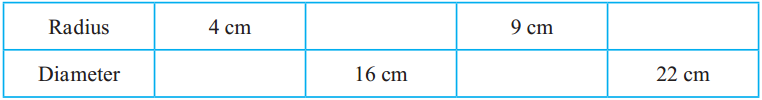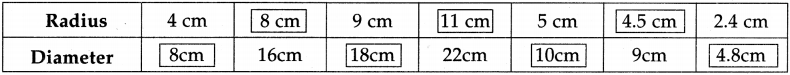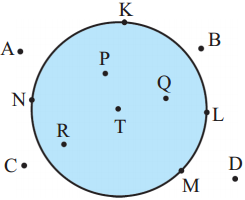# Maharashtra Board Class 5 Maths Solutions Chapter 7 Circles Problem Set 29

Balbharti Maharashtra Board Class 5 Maths Solutions Chapter 7 Circles Problem Set 29 Textbook Exercise Important Questions and Answers.

## Maharashtra State Board Class 5 Maths Solutions Chapter 7 Circles Problem Set 29

Question 1.
If the radius of a circle is 5 cm, what will its diameter be?
Solution :
Diameter
= 2 x 5 = 10 cmQuestion 2.
If the diameter of a circle is 6 cm, what will its radius be?
Solution :
= diameter ÷ 2
= 6 ÷ 2
= 3 cm

Question 3.
Complete the following table by filling in the blanks.The interior and the exterior of a circle

We play ‘Land and Sea’ inside a circle on the playground. In this game, the children inside the circle are in the ‘sea’, while the children outside the circle are on ‘land’.

In the picture alongside, K, L, M and N are points on a circle with centre T.The coloured area inside the circle in the picture is the interior of the circle. P, Q, R and T are points in the interior of the circle.

A, B, C and D are points in the exterior of the circle.

Question 1.
If the radius of a circle is 3.5 cm, what will its diameter be?
Solution :
Diameter